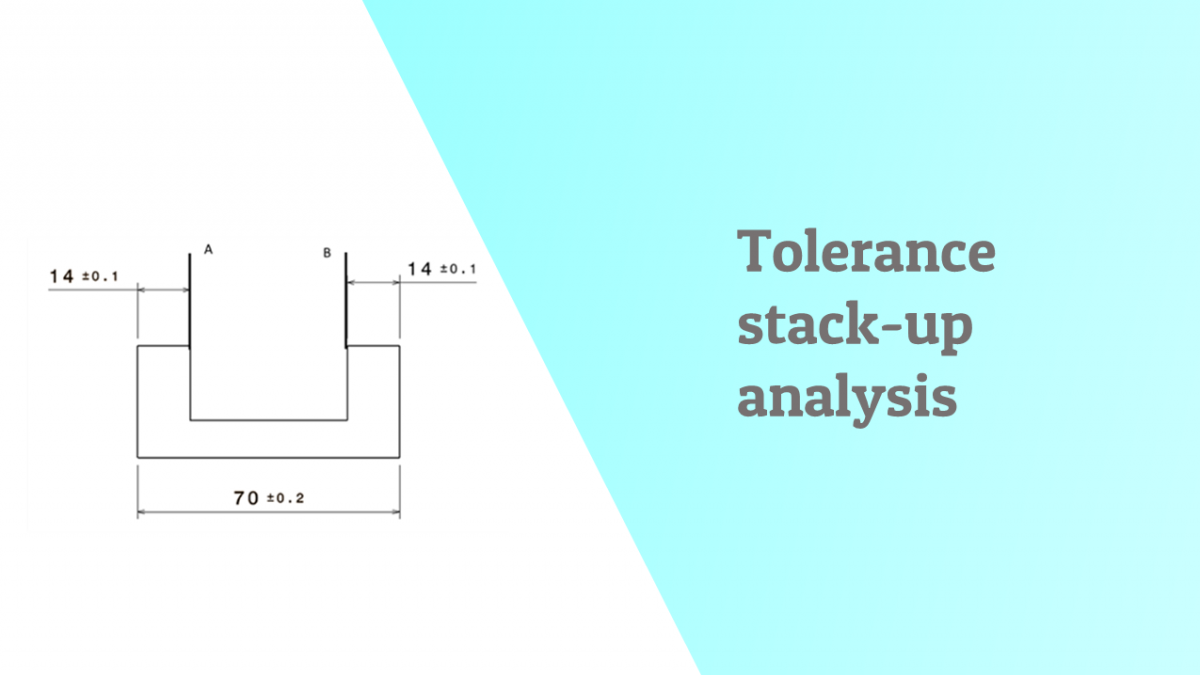# Tolerance stackup analysis of a simple partThis article takes you through a simple example of how tolerance stack up analysis is carried out to find unknown dimensions and gaps

Consider a component as shown with dimensions and tolerances given .

How can we find the specification in red?

Generic process for Worst case tolerance stack up analysis

1. Label the distance to be studied A-B
2. Positive and negative directions are set
1. Left to Right is positive
2. Right to left is negative
3. Develop loop diagram starting from A to B
4. Start from A and move towards B through Loop
5. The dimensions in positive direction are added together and dimensions in negative are added together
6. Negative dimensions sum is subtracted from positive direction sum .
7. All tolerances are added with each other . The total added tolerance is possible allowed variation for distance being studied

Loop Diagram with the tolerance analysis table:

Categories: Tolerancing